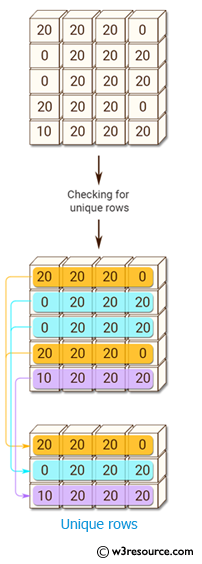﻿ NumPy: Find unique rows in a NumPy array - w3resource# NumPy: Find unique rows in a NumPy array

## NumPy: Array Object Exercise-87 with Solution

Write a NumPy program to find unique rows in a NumPy array.

Pictorial Presentation:Sample Solution:

Python Code:

``````import numpy as np
x = np.array([[20, 20, 20, 0],
[0, 20, 20, 20],
[0, 20, 20, 20],
[20, 20, 20, 0],
[10, 20, 20,20]])
print("Original array:")
print(x)
y = np.ascontiguousarray(x).view(np.dtype((np.void, x.dtype.itemsize * x.shape)))
_, idx = np.unique(y, return_index=True)

unique_result = x[idx]
print("Unique rows of the above array:")
print(unique_result)
```
```

Sample Output:

```Original array:
[[20 20 20  0]
[ 0 20 20 20]
[ 0 20 20 20]
[20 20 20  0]
[10 20 20 20]]
Unique rows of the above array:
[[ 0 20 20 20]
[10 20 20 20]
[20 20 20  0]]
```

Python Code Editor:

Have another way to solve this solution? Contribute your code (and comments) through Disqus.

What is the difficulty level of this exercise?

Test your Python skills with w3resource's quiz

﻿

## Python: Tips of the Day

Set comprehension:

```>>> m = {x ** 2 for x in range(5)}
>>> m
{0, 1, 4, 9, 16}
```